## Heat Transfer Miscellaneous

#### Heat-Transfer

1. Three slabs are joined together as shown in the figure. There is no thermal contact resistance at the interfaces. The cent er slab experience a nonuniform internal heat generation with an average value equal to 10000 Wm-3, while the left and right slabs have no internal heat generation. All slabs have thickness equal to 1 m and thermal conductivity of each slab is equal to 5 Wm-1 K-1. The two extreme faces are exposed to fluid with heat transfer coefficient 100 Wm-2K -1 and bulk temperature 30°C as shown. The heat transfer in the slabs is assumed to be one dimensional and steady, and all properties are constant. If the left extreme face temperature T1 is measured to be 100°C, the right extreme faced temperature T2 is___________°C.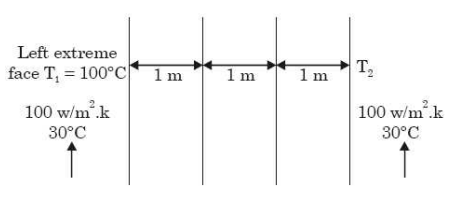1.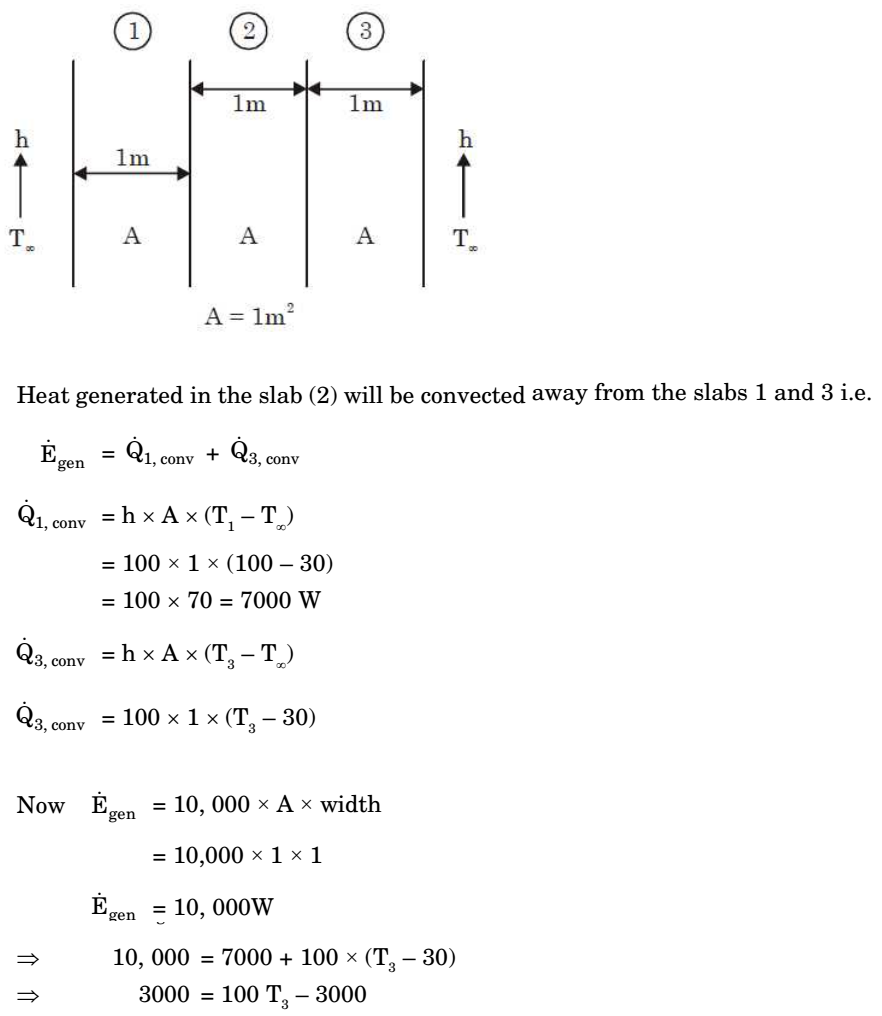##### Correct Option: A1. Heat is generated uniformly in a long solid cylindrical rod (diameter =10 mm) at the rate of 4 × 107 W/m3. The thermal conductivity of the rod material is 25 W/mK. Under steady state conditions, the temperature ditference between the centre and the surface of the rod is 1°C.

1.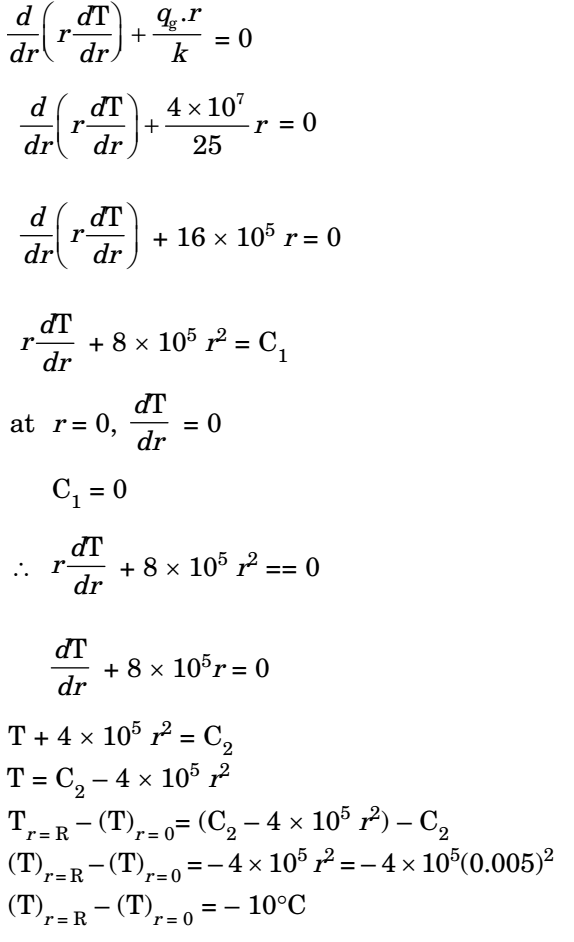##### Correct Option: A1. A cylindrical uranium fuel rod of radius 5 mm in a nuclear is generating heat at the rate of 4 × 107 W/m3. The rod is cooled by a liquid (convective heat transfer coefficient 1000 W/ m2K) at 25°C. At steady state, the surface temperature (in K) of the rod is

1. Given data: r = 0.005 m
d = 2r = 2 × 0.005 = 0.010 m

 q6 = 4 × 107 W m3

 h0 = 1000 W m2K

T0 = 25°c
Rate of heat generation in the rod} = Rate of convection H.T. from rod surface to fluid qG × volume of rod = h0 A(Ts – T0)
 qG × π d²l = h0 × πdl (Ts - T0) 4

 d.qG (Ts - T0) = h0 4

 h0 .010 × 4 × 107 = 1000 (Ts - 25) m24

Ts – 25 = 100
Ts = 125° c or 398 K

##### Correct Option: B

Given data: r = 0.005 m
d = 2r = 2 × 0.005 = 0.010 m

 q6 = 4 × 107 W m3

 h0 = 1000 W m2K

T0 = 25°c
Rate of heat generation in the rod} = Rate of convection H.T. from rod surface to fluid qG × volume of rod = h0 A(Ts – T0)
 qG × π d²l = h0 × πdl (Ts - T0) 4

 d.qG (Ts - T0) = h0 4

 h0 .010 × 4 × 107 = 1000 (Ts - 25) m24

Ts – 25 = 100
Ts = 125° c or 398 K

1. A plane wall has a thermal conductivity of 1.15 W/mK. If the inner surface is at 1100°C and the outer surface is at 350°C, then the design thickness (in meter) of the wall to maintain a steady heat flux of 2500 W/m2 should be

1.  Q = KA dt dx

 q = Q = 2500 W/m² A

 2500 = K 6dt dx

 2500 = 1.15 × = (1100 - 350) x

x = 0.345 m.

##### Correct Option: A

 Q = KA dt dx

 q = Q = 2500 W/m² A

 2500 = K 6dt dx

 2500 = 1.15 × = (1100 - 350) x

x = 0.345 m.

1. A material P of thickness 1 mm is sandwiched between two steel slabs, as shown in the figure below. A heat flux 10 kW/m2 is supplied to one of the steel slabs as shown. The boundary temperatures of the slabs are indicated in the figure. Assume thermal conductivity of this steel is 10 W/mK. Considering one-dimensional steady state heat conduction for the configuration, the thermal conductivity (k, inW/mK) of material P is ________.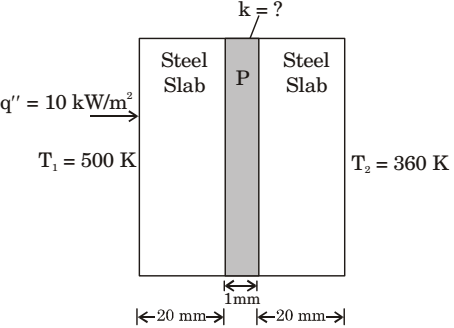1.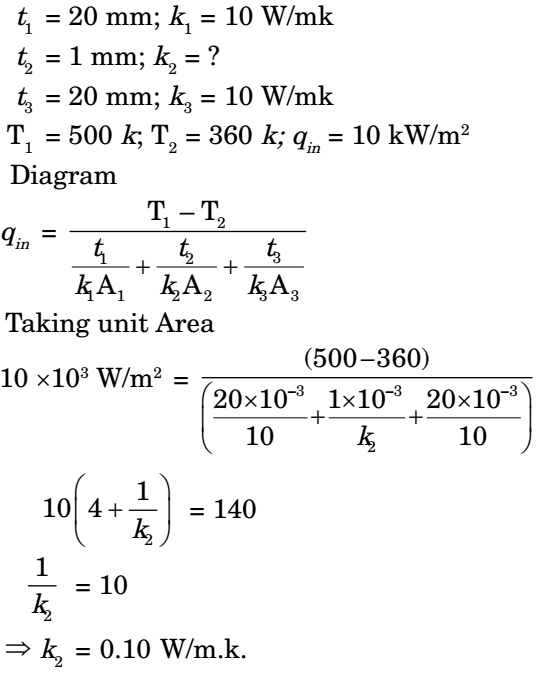##### Correct Option: A